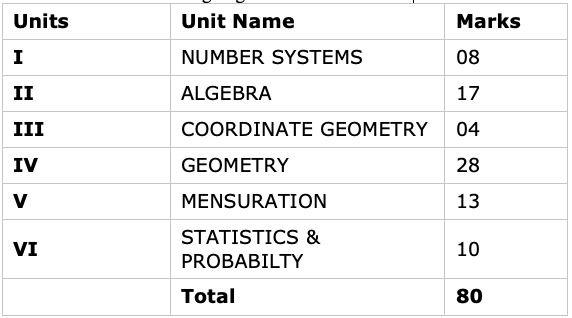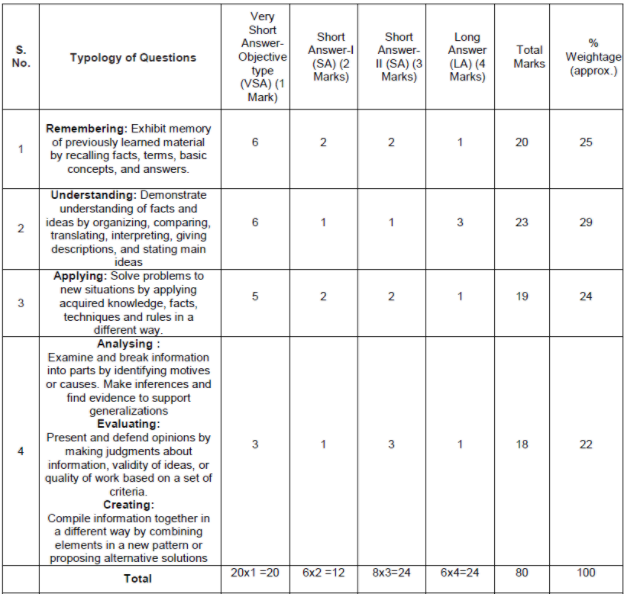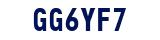# CBSE Sample Papers For Class 9 Maths

## About Sample papers for class 9 Maths

Sample papers for class 9 Maths is prepared by experts of Physics Wallah as per the recommendation of CBSE. Our academic team uploaded more than 20 sample papers for class 9 Maths with solution and few sample papers are without solutions. Solve these sample paper as within time mentioned in each sample paper. If you have any doubt while solving the sample paper post your doubts in ask your doubt section.CBSE Sample Papers class 9 Maths from 1 to 5 consist of all types of questions and than after all sample papers consist of advance questions based on class 9 Maths and all are MCQ based for complete revision. Do solve questions given in NCERT exercise with the help of Physics Wallah NCERT Solutions for class 9 Maths.## Find Below Sample papers for class 9 Maths for reference

### Understand the pattern of class 9 Maths

1. Total Marks of Class 9 Maths Exam is = 100 marks
2. Out of which 80 marks are assigned to the theory paper and rest 20 marks are kept for internal assessment.
3. Internal assessment will comprise following learning activities:
• (i) Pen Paper Test and Multiple Assessment = 5+5 = 10 Marks
• (ii) Portfolio = 05 Marks
• (iii) Lab Practical (Lab activities) = 05 Marks

### Understand the unit wise weightage of CBSE Class 9 Maths Sample papers

Before solving Sample papers for class 9 Maths one must understand the Maths question. Class 9 maths paper in the annual exams will be prepared according to the unit-wise weightage distribution as prescribed by CBSE in the Class 9 Maths Syllabus for the current session.For more sample papers check out Physics Wallah.

list of units with their weightage for the annual exam### Sample papers for class 9 Maths design will be as per the following scheme

The class 9 Maths test paper will comprise of a total of total 40 questions divided into four sections, such as A, B, C and D sections.

• Section A will consist of  20 questions of 1 mark each.
• Section B will consist of 6 questions of 2 marks each.
• Section C will consist of 8 questions of 3 marks each.
• Section D will consist of 6 questions of 4 marks each.

Objective type questions are framed in different types like MCQ based and fill in the blanks and short answer type questions.Students can also get class 9 maths notes at Physics Wallah and they can also check their skills and understanding towards the subject by taking Maths Quiz class 9

### FAQ FOR CBSE SAMPLE PAPERS CLASS 9 MATHS

Q1. How CBSE sample papers are useful for class 9 maths?

Ans. CBSE sample papers are the best resource for class 9 math as through it students can understand the pattern of exam question papers. By solving these CBSE sample papers, students have a good idea of what to expect during the exam. They also know the questionnaire model, the weight of the questions in each section, and the evaluation scheme. So to help prepare students, we have provided class 9 math CBSE sample papers.

Q2. What are CBSE class 9 maths sample papers?

Ans. CBSE Class 9 math is a school exam, so different schools organize different questionnaires. There is no common questionnaire for all class 9 student schools. Therefore, it is difficult to obtain last year's class 9 math questionnaires. However, students can easily find CBSE Class 9 math questionnaires online and offline for practice.

Q3 Which is the best site for class 9 maths sample papers?

Ans. Students can find class 9 math CBSE sample papers in PDF download format. At Physics Wallah we provide sample papers for class 9 mathematics with solutions by our academic expert teachers. Sample papers for summative assessment 1 and 2 were also provided by students for practice. Students can download math class 9 sample papers by our site.

Q4 How many sample papers should be practiced for the CBSE class 9 maths?

Ans. It depends on whether your preparation is good enough and you have completed the whole syllabus of class 9 maths and if you are confident enough in almost the topics, you don't have to solve many sample papers. But if some topics are good and you're not sure about other topics, you need to solve 7-9 papers so that you can build confidence for the exam.

Q5 What are the benefits of practicing CBSE Sample papers for class 9 math?

Ans. Each class 9 students will gain multiple benefits by practicing CBSE sample papers for class 9 maths. With the help of these paper sample sets, you can easily improve your exam preparation. In this way, you can also improve the topics in which you are weak, such as algebra, probability, trigonometry or geometry, etc. The best advantage you can get from this is that you can improve your time management skills.

Q6 Does Physics Wallah provide solutions for the CBSE sample papers for class 9 maths?

Ans. Yes, Physics Wallah offers solutions for CBSE sample papers for class 9 mathematics. You can access them through our website or mobile app. These are available in PDF format for easy download. Another advantage is that they are free. All of these solutions have been put together by our excellent subject matter experts, so you can refer to them without a doubt.